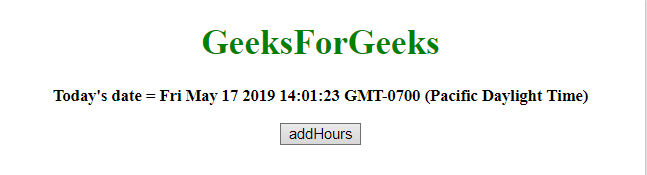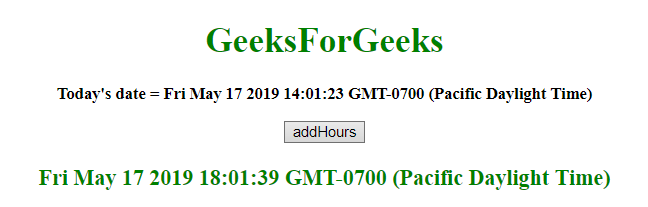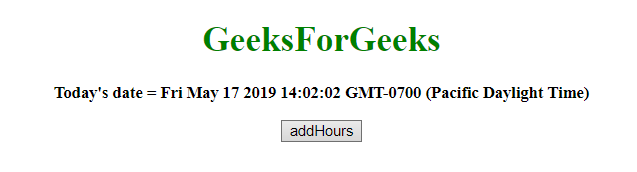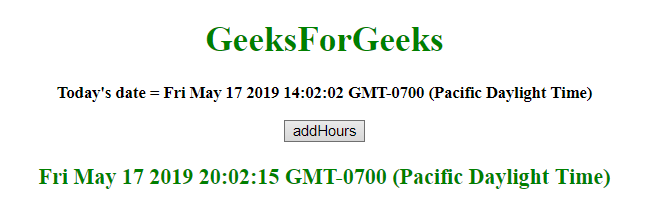# JavaScript | Adding hours to the Date object

Given a date, the task is to add hours to it. To add hours to date in javascript, we’re going to discuss a few techniques. First few methods to know.

• JavaScript getHours() Method
This method returns the hour (from 0 to 23) of the provided date and time.
Syntax:

```Date.getHours()
```

Return value:
It returns a number, from 0 to 23, representing the hour.

• JavaScript setHours() Method
This method sets the hour of a date object.
We can also set the minutes, seconds and milliseconds.
Syntax:

```Date.setHours(hour, min, sec, millisec)
```

Parameters:

• hour: This parameter is required. It specifies the integer representing the hour. Values expected are 0-23, but other values are allowed.
• min: This parameter is optional. It specifies the integer representing the minutes. Values expected are 0-59, but other values are allowed.
• sec: This parameter is optional. It specifies the integer representing the seconds. Values expected are 0-59, but other values are allowed.
• millisec: This parameter is optional. It specifies the integer representing the milliseconds. Values expected are 0-999, but other values are allowed.

Note:
All the previous 4 parameters accept values apart from their range and these values adjust like.

• hour = -1, means the last hour of the previous day and same for the other parameters.
• if min passed is 60, means the first minute of the next hour and same for the other parameters.

Return value:
It returns a number, denoting the number of milliseconds between the date object and midnight January 1, 1970.

• JavaScript getTime() method
This method returns the number of milliseconds between midnight of January 1, 1970, and the specified date.
Syntax:

```Date.getTime()
```

Return value:
It returns a number, representing the number of milliseconds since midnight January 1, 1970.

• JavaScript setTime() method
This method set the date and time by adding/subtracting a defines number of milliseconds to/from midnight January 1, 1970.
Syntax:

```Date.setTime(millisec)
```

Parameters:

• millisec: This parameter is required. It specifies the number of milliseconds to be added/subtracted, midnight January 1, 1970

Return value:
It returns, representing the number of milliseconds between the date object and midnight January 1 1970.

• Example 1: This example adds 4 hours to the 16th may by using setTime() and getTime() method.

 ` ` `<``html``> ` ` `  `<``head``> ` `    ``<``title``> ` `        ``JavaScript  ` `      ``| Adding hours to Date object. ` `    `` ` ` ` ` `  `<``body` `style``=``"text-align:center;"`  `      ``id``=``"body"``> ` `    ``<``h1` `style``=``"color:green;"``>   ` `            ``GeeksForGeeks   ` `        `` ` `    ``<``p` `id``=``"GFG_UP"`  `       ``style="font-size: 15px; ` `              ``font-weight: bold;"> ` `    `` ` `    ``<``button` `onclick``=``"gfg_Run()"``> ` `        ``addHours ` `    `` ` `    ``<``p` `id``=``"GFG_DOWN"`  `       ``style="color:green;  ` `              ``font-size: 20px;  ` `              ``font-weight: bold;"> ` `    `` ` `    ``<``script``> ` `        ``var el_up =  ` `            ``document.getElementById("GFG_UP"); ` `       `  `        ``var el_down =  ` `            ``document.getElementById("GFG_DOWN"); ` `        ``var today = new Date(); ` `        ``el_up.innerHTML = "Today's date = " + today; ` `        ``Date.prototype.addHours = function(h) { ` `            ``this.setTime(this.getTime() + ` `                         ``(h * 60 * 60 * 1000)); ` `            ``return this; ` `        ``} ` ` `  `        ``function gfg_Run() { ` `            ``var a = new Date(); ` `            ``a.addHours(4); ` `            ``el_down.innerHTML = a; ` `        ``} ` `    `` ` ` ` ` `  ` `

Output:

• Before clicking on the button:• After clicking on the button:Example 2: This example adds 6 hours to the 16th may by using setHours() and getHours() method.

 ` ` `<``html``> ` ` `  `<``head``> ` `    ``<``title``> ` `        ``JavaScript  ` `      ``| Adding hours to Date object. ` `    `` ` ` ` ` `  `<``body` `style``=``"text-align:center;"`  `      ``id``=``"body"``> ` `    ``<``h1` `style``=``"color:green;"``>   ` `            ``GeeksForGeeks   ` `        `` ` `    ``<``p` `id``=``"GFG_UP"`  `       ``style="font-size: 15px;  ` `              ``font-weight: bold;"> ` `    `` ` `    ``<``button` `onclick``=``"gfg_Run()"``> ` `        ``addHours ` `    `` ` `    ``<``p` `id``=``"GFG_DOWN"`  `       ``style="color:green;  ` `              ``font-size: 20px; ` `              ``font-weight: bold;"> ` `    `` ` `    ``<``script``> ` `        ``var el_up =  ` `            ``document.getElementById("GFG_UP"); ` `        ``var el_down =  ` `            ``document.getElementById("GFG_DOWN"); ` `        ``var today = new Date(); ` `        ``el_up.innerHTML = "Today's date = " + today; ` `        ``Date.prototype.addHours = function(h) { ` `            ``this.setHours(this.getHours() + h); ` `            ``return this; ` `        ``} ` ` `  `        ``function gfg_Run() { ` `            ``var a = new Date(); ` `            ``a.addHours(6); ` `            ``el_down.innerHTML = a; ` `        ``} ` `    `` ` ` ` ` `  ` `

Output:

• Before clicking on the button:• After clicking on the button:My Personal Notes arrow_drop_upCheck out this Author's contributed articles.

If you like GeeksforGeeks and would like to contribute, you can also write an article using contribute.geeksforgeeks.org or mail your article to contribute@geeksforgeeks.org. See your article appearing on the GeeksforGeeks main page and help other Geeks.

Please Improve this article if you find anything incorrect by clicking on the "Improve Article" button below.

Article Tags :

Be the First to upvote.

Please write to us at contribute@geeksforgeeks.org to report any issue with the above content.